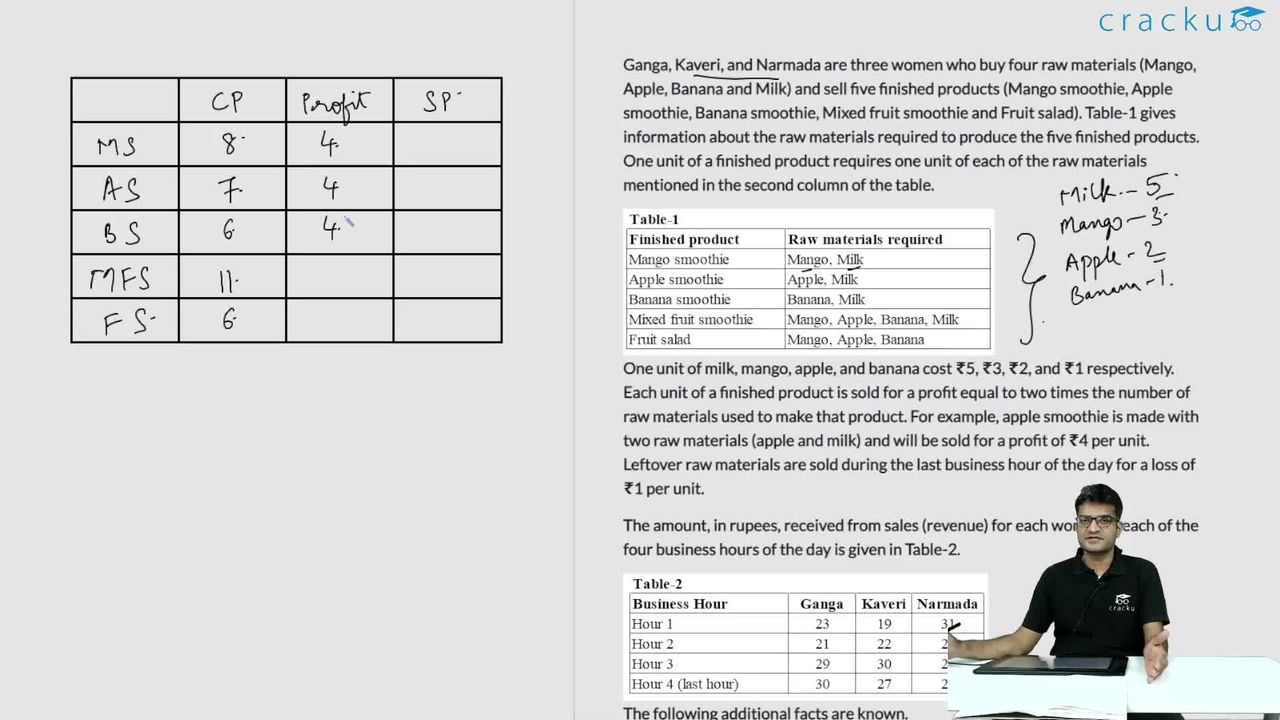### CAT 2021 Question Paper (Slot 1) Question 34

Instructions

Ganga, Kaveri, and Narmada are three women who buy four raw materials (Mango, Apple, Banana and Milk) and sell five finished products (Mango smoothie, Apple smoothie, Banana smoothie, Mixed fruit smoothie and Fruit salad). Table-1 gives information about the raw materials required to produce the five finished products. One unit of a finished product requires one unit of each of the raw materials mentioned in the second column of the table.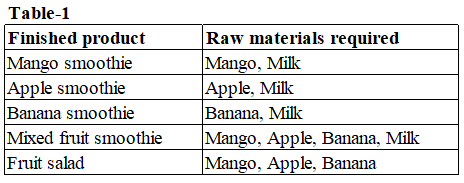One unit of milk, mango, apple, and banana cost ₹5, ₹3, ₹2, and ₹1 respectively. Each unit of a finished product is sold for a profit equal to two times the number of raw materials used to make that product. For example, apple smoothie is made with two raw materials (apple and milk) and will be sold for a profit of ₹4 per unit. Leftover raw materials are sold during the last business hour of the day for a loss of ₹1 per unit.

The amount, in rupees, received from sales (revenue) for each woman in each of the four business hours of the day is given in Table-2.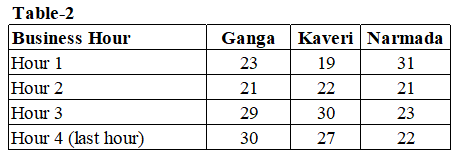The following additional facts are known.
1. No one except possibly Ganga sold any Mango smoothie.
2. Each woman sold either zero or one unit of any single finished product in any hour.
3. Each woman had exactly one unit each of two different raw materials as leftovers.
4. No one had any banana leftover.

Question 34

# If it is known that three leftover units of mangoes were sold during the last business hour of the day, how many apple smoothies were sold during the day?

Solution

Given that each item is sold for a profit of 2 times the number of materials required for the dish.

Hence for different finished products: The cost price and selling price are :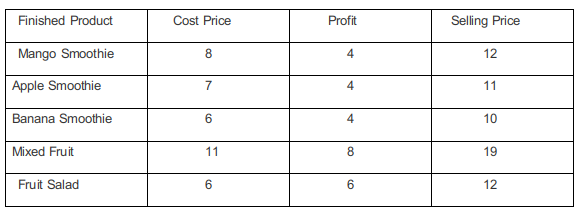Given that in any hour only either zero or one unit of a single finished product is sold. Hence the price distribution for the first three hours sales distribution is given by :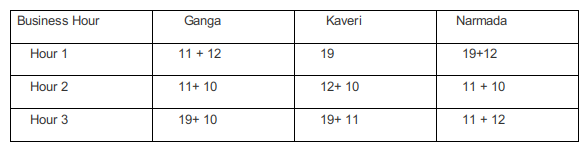Additionally, it has been mentioned that no one except Ganga possibly sold Mango smoothies.

Considering Apple Smoothie : (AS), Mango Smoothie : ( M.S), Banana Smoothie : (B. S), Mixed Fruit : (M.F), Fruit Salad : (F.S)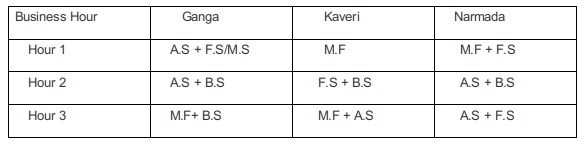In the last one hour everyone had one unit each of two different materials as leftovers:

Each leftover item is sold at Re1 less than their cost price. Hence

One unit of milk, mango, and apple will cost ₹4, ₹2, ₹1,

The three possible combinations for the last hour in which raw materials can be sold is :

One unit of milk+ One unit of mango = Rs 4+2 = Rs 6.

One unit of milk+ One unit of apple = Rs 4+1 = Rs 5.

One unit of mango+ One unit of apple = Rs 2+1 = Rs 3.

In the last one hour :

Total sale of Ganga is Rs 30. This is the total cost earned by considering selling the finished items and raw materials :

Hence the possible cases are :

(24 + 6) or (25 + 5) or ( 27 + 3). It is not possible to earn 25 and 27 by selling finished products.

Hence she earns Rs 24 which is possible by selling one ( M.S + F.S) and one raw unit of milk and mango each.

Total sale of Kaveri is Rs 27. This is the total cost earned by considering selling the finished items and raw materials :

Hence the possible cases are :

(21 + 6) or (22+5) or (24+3).

Since Kaveri cannot sell mango smoothies the possible cases are :

(21+6) Selling one apple smoothie and one banana smoothie and one raw unit of Milk and Mango.

(22+5) Selling one Banana smoothie and Fruit Salad, one unit of raw milk, and one unit of Raw Apple.

Total sale for Narmada is Rs 22. The possible cases are :

(16+6), (17+5), (19+3).

Among these, the only possible case is 19 + 3. Selling one unit Mixed Fruit, and one raw unit of Mango and Apple.

Case 1 :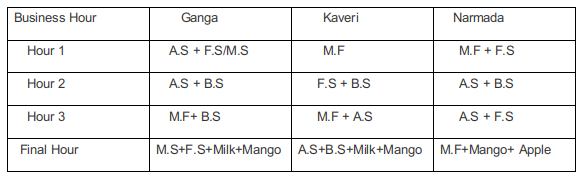case 2 :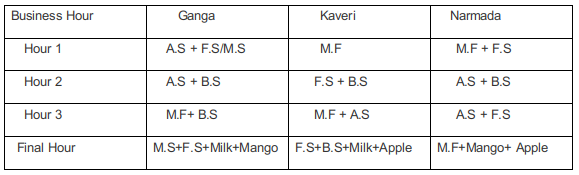3 units of leftover mangoes were available in case 1 and in here a total of 6 apple shakes were sold.

### View Video Solution